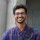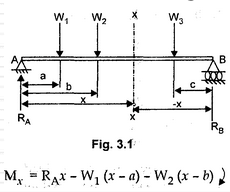Coffee Room
Discuss anything here - everything that you wish to discuss with fellow engineers.
12940 Members
Join this group to post and comment.Ankush Sharma • Dec 19, 2012

# Doubts regarding Strength of material

hi friends...... i have few doubts regarding strength of material, so please can anyone give me solution for them........
1. why stress decreases from upper yield point to lower yield point in stress-strain curve?
2. when we draw shear force diagram and bending moment diagrams for beams, we usually see that when shear force is zero at that time bending moment is maximum, so my question is,why?
3. what is difference between moment of inertia and polar moment of inertia?Ambarish Ganesh • Dec 19, 2012
Consider this-While M is the bending moment, the Shear Force F= Ra-W1-W2

now, when F=0, Ra=W1+W2, which when substituted in M gives you the maximum bending moment, i.e. M=W1a+W2b.

Ok, now let's see why this is maximum. See that the last equation contains no variable (no 'x'), so the rate of change of moment (dM/dx) must be zero for maximum bending moment right? 😀 Now as Moment is constant, differentiating it gives zero. Hence maximum bending moment.Ankush Sharma • Dec 20, 2012
The_Wise_Fool
Consider this-While M is the bending moment, the Shear Force F= Ra-W1-W2

now, when F=0, Ra=W1+W2, which when substituted in M gives you the maximum bending moment, i.e. M=W1a+W2b.

Ok, now let's see why this is maximum. See that the last equation contains no variable (no 'x'), so the rate of change of moment (dM/dx) must be zero for maximum bending moment right? 😀 Now as Moment is constant, differentiating it gives zero. Hence maximum bending moment.
ok dear, right description................ but it is numerical one................ but, what if i suddenly ask this question from u?????? means if this question is asked in interview from u, then at that time what will u say?????? waiting...........